# GMAT Sample Data Sufficiency Practice Questions

Unlike Problem Solving, Data Sufficiency is a question-type unique to the GMAT.  Data Sufficiency questions demand their own logic and their own strategies.

## Sample Data Sufficiency questions from the blog

Below are links to seven different blog articles, each with at least two Data Sufficiency sample questions.   The solutions are given at the end of each article.

Four practice DS questions on Geometric Solids

https://magoosh.com/gmat/math/geometry/gmat-math-3d-solids/

Two practice DS questions involving variables & Data Sufficiency

https://magoosh.com/gmat/math/data-sufficiency/gmat-data-sufficiency-strategies/

Six practice DS questions on what determines a square

https://magoosh.com/gmat/math/geometry/gmat-geometry-is-it-a-square/

Two practice DS questions on the properties of the line y = x

https://magoosh.com/gmat/math/geometry/gmat-math-special-properties-of-the-line-y-x/

Three practice DS questions on exponents and powers

https://magoosh.com/gmat/math/arithmetic/exponent-powers-on-the-gmat/

Two practice DS questions on roots

https://magoosh.com/gmat/math/basics/gmat-quant-roots/

Two practice DS questions on fractions

## New Data Sufficiency practice questions

Here are 12 questions, created fresh, specifically for this blog article.  Answers and explanations appear at the end of this article.

1) P and Q are positive integers.  Is P odd?

Statement #1: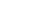is odd

Statement #2: P + Q is even

2) The numbers a, b, c, and d are all positive, and J = a/b and K = c/d.  Is J greater than K?

Statement #1: a < b, c < d, and c > a

Statement #2: a + 7 = c and b + 4 = d

3) What is the value of x?

Statement #1: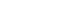Statement #2: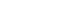4) Rachel drove the 120 miles from A to B at a constant speed.  What was this speed?

Statement #1: If she had driven 50% faster, her new time would have by 2/3 of her original time.

Statement #2: If she drove 20 mph faster, she would have arrived an hour sooner.

5) Suppose n children are going to sit, in any order, on a row of n chairs.  What is the value of n?

Statement #1: In this row, there are 5040 possible arrangements of the n children.

Statement #2: If one child leaves, and one chair is removed from the row, there would be 4320 fewer arrangements of the remaining children.

6)  What is the value of x?

Statement #1: 6x – 14y = 10

Statement #2: 21y = 9x – 15

7) What is the value of x?

Statement #1: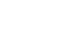Statement #2: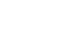8) In the diagram above, ∠A = ∠F = 50°, ∠B = 20°, and ∠H = 110°.  What is the length of FG?

Statement #1: triangle FGH has 9 times the area of triangle ABC

Statement #2: HF = 99) In the diagram above, what is the circumference of the circle?

Statement #1: The area of triangle is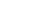Statement #2: ∠B = 60°

10) Line L is a line in the x-y plane.  Does line L pass through the second quadrant?

Statement #1: Line L has a positive slope

Statement #2: At its closest approach, Line L is never closer than 3 units to the origin.

11) Throughout last year, a stylish jacket had a full price of P in certain store.  At the beginning of this year, the regular full price of the jacket rose A% percent.  Shortly after the beginning of this year, this store had a sale, and Margarette bought the jacket at B% less than then this year’s current full price, where B > A.  How much did Margarette save, buying the jacket this year on sale, as compared to what she would have spent last year?

Statement #1: P = \$250

Statement #2: B – A = 5

12) The numbers a, b, and c are all positive.  If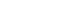, then what is the value of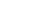?

Statement #1: a – b = 3

Statement #2: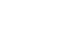1) A

2) C

3) B

4) B

5) D

6) E

7) A

8) D

9) C

10) E

11) E

12) C

## Explanations for the 12 new DS questions

1) P and Q are positive integers.  Is P odd?

Statement #1:is odd

The only way a product can be odd is if each of the factors is odd; therefore, ifis odd, then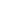and Q each must be odd.  The only way the square of a positive integer can be odd is if the original integer is odd: therefore, if, the P must be odd.  This statement, alone and by itself, is sufficient to give a definitive “yes” answer to the prompt question.

Statement #2: P + Q is even

Here, both P & Q together can be even, or both P & Q together can be odd.  On the basis of this statement alone, which don’t know which of those two possibilities is the case.  Therefore, we are no position to give a definitive answer to the prompt question.  This statement, by itself, is insufficient.

2) The numbers a, b, c, and d are all positive, and J = a/b and K = c/d.  Is J greater than K?

Statement #1: a < b, c < d, and c > a

The first two inequalities tells us that both J and K are fractions less than one.  From this and third inequality, we can draw no conclusion about which is bigger.  For example, J = 9/10 and K = 11/50 satisfy all three conditions, and J > K, while J = 7/100 and K = 9/00 satisfy all three conditions, and J < K.  This statement can produce either answer to the prompt question.  Therefore, this statement, alone and by itself, is insufficient.

Statement #2: a + 7 = c and b + 4 = d

We need to forget everything associated with statement #1.  Now we are focused purely on the issue of adding to the numerator and the denominator.  Understanding this question depends very much on the concept discussed in that blog article.   As you will see in that post, adding 7 to the numerator and 4 to the denominator will have the net effect of moving the fraction closer to 7/4 on the number line.  If the original fraction is less than 7/4, for example, J = 1/2, then this addition, in moving the fraction closer to 7/4, makes the fraction bigger — K = 7/6 is greater than J = 1/2.  BUT, if the original fraction is more than 7/4, for example, J = 25/4 = 6.25, then this addition, in moving the fraction closer to 7/4, makes the fraction smaller — K = 32/8 = 4 is less than J = 1/2.  This statement can produce either answer to the prompt question.  Therefore, this statement, alone and by itself, is insufficient.

Statements #1 & #2 combined:

The first statement tells us that J < 1 and K < 1.  The second statement, in adding 7 to the numerator and 4 to the denominator will have the net effect of moving the K closer than J to 7/4 on the number line.  Any fraction that is less than one gets bigger if it moves closer to 7/4.  Therefore, J < K.  Combined, the statements are sufficient.

3) What is the value of x?

Statement #1:A positive squared is positive, and negative squared is also positive, so here x could be +10 or –10.  Because we don’t know which of these is x, we can’t determine a unique answer to the prompt question.  Therefore, this statement, alone and by itself, is insufficient.

Statement #2:A positive cubed is positive, but a negative cubed is negative.  Because the cube, 1000, is positive, it means x must equal +10.  This statement allows us to obtain a definitive answer to the prompt question.  This statement, alone and by itself, is sufficient.

4) Rachel drove the 120 miles from A to B at a constant speed.  What was this speed?

Let’s say the original variables are D = 120, R, and T, and the original case is 120 = RT.

Statement #1: If she had driven 50% faster, her new time would have by 2/3 of her original time.

To increase by 50%, we will multiply by the multiplier 1.5.   This means that the new speed, R2, is R2 = 1.50*R = (3/2)*R.  The new time is T2 = (2/3)*T.  Well, we know, that 120 = (R2)*(T2) — the new speed and time must have the small product as the original speed and time.  120 = (R2)*(T2) = [(3/2)*R]*[(2/3)*T] = (3/2)*(2/3)*RT = RT.  This information leads in a big logical circle right back to the original equation 120 = RT.  It gives us no new information at all.   Therefore, this statement, by itself, does not provide any insight into the answer to the prompt question.  This statement is insufficient.

Statement #2: If she drove 20 mph faster, she would have arrived an hour sooner.

Now, we know R2 = R + 20 and T2 = T – 1.  Again, we know that 120 = (R2)*(T2), so

120 = (R + 20)*(T – 1) = RT – R + 20T – 20

120 – RT = 0 = 20T – R – 20

That is one equation with two unknowns, and 120 = RT is a second equation with two unknowns.  We have two clearly different equations for the two unknowns, so even without solving, this is sufficient to determine a unique value for the variables R & T.

While it’s not at all necessary to answer the DS question for those who would like to see the solution of the equations with two unknowns, here it is:Multiply all three terms by T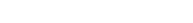Put the terms in order of powers of T, and divide both sides by the GCF, which is 20We know that both R and T must be positive, so this gives us values of T = 3 hours and R = 40 mph.  If Rachel drove faster, R2 = 60 mph, then should would have arrived in T2 = 2 hrs, one hour sooner.  This answer checks.

5) This is a question about permutations.   Because this is Data Sufficiency, we don’t have to bother with the details of calculating n! —- it’s enough to know that if we know the value of n, we can figure out the value of n!, and vice versa!

Statement #1: this gives us n! = 5040 — from this we could figure out n.  Statement #1, alone and by itself, is sufficient.

Statement #2: this tells us, essentially, n! – (n – 1)! = 4320.  Well, if we went through list of factorials, there could be only one pair that is separated by exactly 4320.  We don’t have to know what it is — it’s enough to know that this fact determines a unique value of n.   Statement #2, alone and by itself, is sufficient.

(BTW, n = 7 if you’re curious!)

6) Statement #1: one equation, two variables.  Statement #1, alone and by itself, is insufficient.

Statement #2: one equation, two variables.  Statement #2, alone and by itself, is insufficient.

Statements #1 & #2 combined: ordinarily, two equations with two variables would be sufficient — if in fact we have two different equations.  Notice though: divide the second equation by 3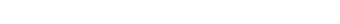Now, divide the first equation by 2 and get the y-term on one side by itself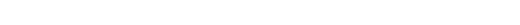We don’t have two different equations after all!  We have the same equation in two different forms!  We are still in the situation of: one equation, two variables.   Even combined, the statements are insufficient.

7) Statement #1: this statement must mean that (x – 5) = 0, which means x = 5.  This determines a unique value for x.  Statement #1, alone and by itself, is sufficient.

Statement #2: this statement must mean that (x – 3) = ±2, which leads to two possible values for x.  This does not determine a unique and definitive value, so statement #2, alone and by itself, is insufficient.

8) The information given implies that ∠C = 110° and ∠G = 20°, because the sum of the three angles in each triangle must be 180°, by Euclid’s well-known theorem.   Thus, the two triangles have all the same angles, but they are different sizes — they are similar.  We know the length of AB, so all we need is the scale factor to determine length of the corresponding side FG.

Statement #1: this statement tells us the ratio of areas is 9 —- this is the square of the scale factor, so k = 3, and from this we can calculate the length of FG.   Statement #1, alone and by itself, is sufficient.

Statement #2: this statement gives us a third side, so we can set up a proportion: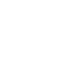Since we now know three of the terms of that proportion, we can solve for the fourth, FG.  Statement #2, alone and by itself, is sufficient.

9) From the diagram, we know that BC is a diameter — the special case of a triangle in a semicircle.  If we can find BC, we can find the circumference.

Statement #1: this gives us the area of the triangle, but the angles could be anything, and so the sides could have an array of different values.   Statement #1, alone and by itself, is insufficient.

Statement #2: this statement would allow us to figure out all three angles of triangle ABC, but you need a length to find a length.  Without any length given, we can’t find the length of BC or the circumference. Statement #2, alone and by itself, is insufficient.

Statements #1 & #2 combined: Now, with both statements, we know ABC is a 30-60-90 triangle, and we know its area, so we could figure out the side lengths, which would allow us to figure out the diameter & circumference.   Combined, the statements are sufficient.

10) Statement #1: Line L has a positive slope

Case one — line below origin goes through quadrants III, IV, and I.Case two — line through origin goes through just quadrants III & ICase three — line below origin goes through quadrants III, II, and I.Some lines with positive slopes go through Quadrant II, and some do not.  Statement #1, alone and by itself, is insufficient.

Statement #2: At its closest approach, Line L is never closer than 3 units to the origin.

In the diagram below, the red circle has a radius of three, and no line crosses within this circle — thus, every line in this diagram meets the condition given in Statement #2.All lines with negative slopes pass through Quadrant II.  Vertical lines pass through Quadrant II only if they are to the left of the y-axis.  Horizontal lines pass through Quadrant II only if they are above the x-axis.  As we saw in statement #1, some lines with positive slope pass through Quadrant II and some don’t.  Statement #2, alone and by itself, is insufficient.

Statements #1 & #2 combined: Now, we are confined to lines with positive slopes and we also have the red circle.   The following diagram tells the whole story:Both of these lines meet both conditions — positive slopes and outside the red circle.  One of them passes through Quadrant II, and one of the them doesn’t.  Therefore, even if we know both conditions are true, that will not answer the prompt question.  Combined, the statements are still insufficient.

11) This is a question that plays on a very specific math mistake.   Most people will get, with relatively little effort, that the individual statements are not sufficient on their own.  Let’s jump ahead to the point at which we are assuming both statements are true.  P = \$250, and B – A = 5.  Now what?

Here is the BIG MISTAKE.  People think if a percent P increase is followed by a percent Q decrease, the net change in percent is P – Q.  This might be the single deadliest trap on the entire Quant section.  All the test writers have to do is set up a situation which elicits this assumption, then they can just sit back in chaise lounges, sipping lemonade, and watch hordes of GMAT test takers run into the big butterfly net.  Don’t be one of the lemmings!  Don’t be fooled!  If nothing else, learn that for a series of percent changes, it is always guaranteed wrong 100% of the time to add & subtract the percentages directly.  Even if you don’t learn the correct procedure, at least learn that this is wrong.

The correct thing to do is to use multipliers for percentage increase & decrease.   Here are a couple extreme examples.  Example #1 — let’s say A = 0%, no increase.  Last year price = \$250, and this year’s price is also \$250.  Then B = A + 5 = 5%, so the sale price is 5% lower, for a savings of \$12.50.  Example #2 — let’s say A = 95%.  Last year price = \$250, and this year’s price is something much bigger, closer to \$500, we don’t care exactly what.  Now, B = A + 5 = 100%, so the entire price is deducted, to a new price of \$0 — they simply give the jacket away.  That’s a savings of \$250 on a \$250 jacket — not bad at all!  The point is, though, we could construct two different examples with two different dollar amounts saved.  This means, even with both statements, we cannot give a definitive answer to the prompt question.  Combined, the statements are still insufficient.

12) Let X =, the value we are seeking. Notice if we subtract the first equation in the prompt from this equation, we get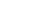= X – 17.  In other words, if we could find the value of, then we could find the value of X.

Statement #1: a – b = 3

From this statement alone, we cannot calculate, so we can’t find the value of X.  Statement #1, alone and by itself, is insufficient.

Statement #2:From this statement alone, we cannot calculate, so we can’t find the value of X.  Statement #2, alone and by itself, is insufficient.

Statements #1 & #2 combined: Now, if we know both statements are true, then we could multiple these two equations, which cancel the denominator, and result in the simple equation a + b = 21.  Now, we have the numerical value of both (a – b) and (a + b), so from the difference of two squares formula, we can figure out, and if we know the numerical value of that, we can calculate X and answer the prompt.   Combined, the statements are sufficient.

•